Tag ctex, 排版, on by view 7583

• latex工具选择

• 包选择

• 编辑器

• 书籍结构

ctexbook中，一般有如下结构：chapter（章）, section（节）, subsection（子节）, subsubsection（三级子节）, paragraph（段落）, subparagraph（子段落）等，用得最多的是前五种。最基础的文章结构为段落，分段常用空行的方式进行，之前我误以为 \paragraph{内容} 是分段的方式，结果发现段落全部默认粗体字了，后来才明白，\paragraph{} 应当作为“段落标题”或是成为“段落首句加粗”，其起着重作用。上一层结构就是三级子节了，如果书籍中没有那么细的分节，三级子节可以不要，三级子节默认加粗，左对齐，\subsubsection{三级子节} 被\subsubsection命令括起来的部分就是三级子节的标题了，如下图；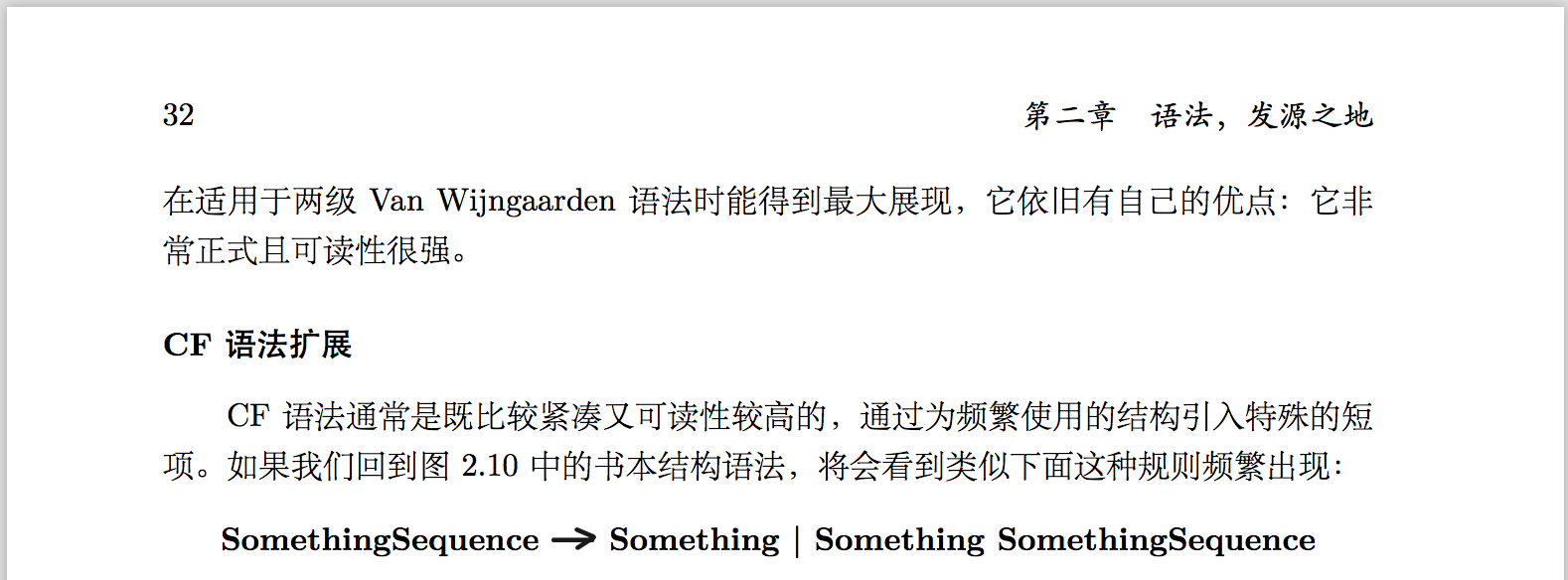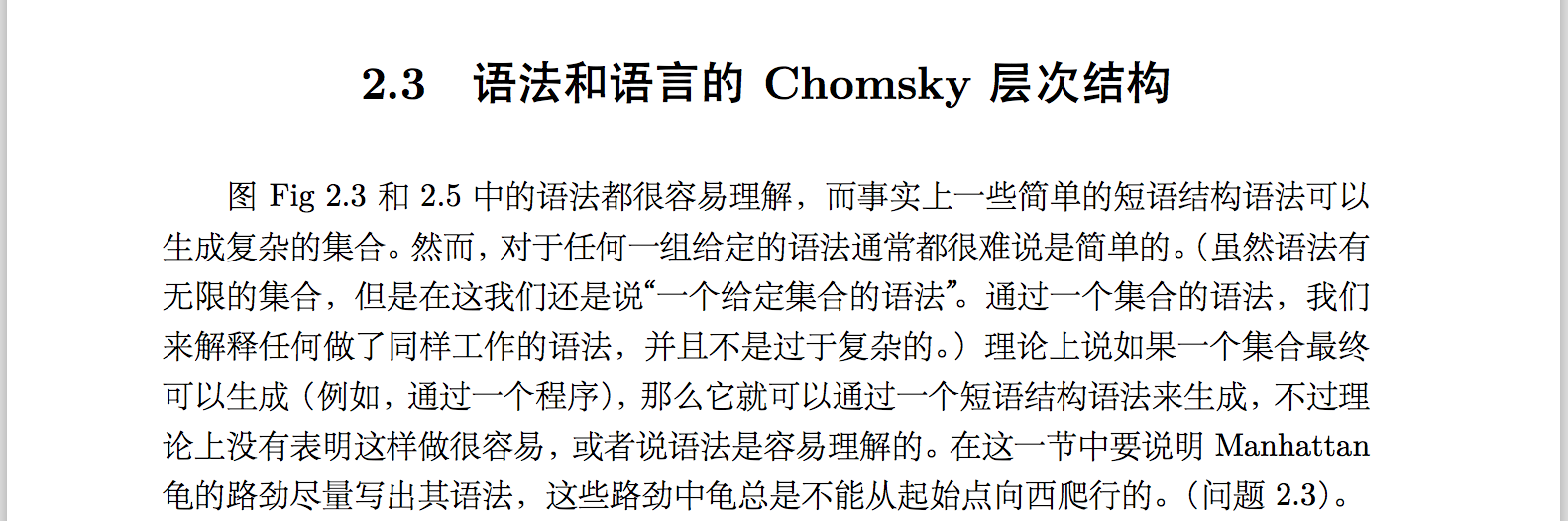• 内容结构

\documentclass[hyperref,UTF8]{ctexbook}
% \documentclass{amslatex}
\usepackage{src/pt}

\begin{document}

% \pagestyle{plain}

% 前言开始
\frontmatter

\include{data/preface2}
\include{data/preface1}

% 正文开始
\mainmatter

\tableofcontents

\include{data/chap1}
\include{data/chap2}

% 尾言开始
\backmatter

\end{document}
• 前言中定义章节\section*{关于练习和问题}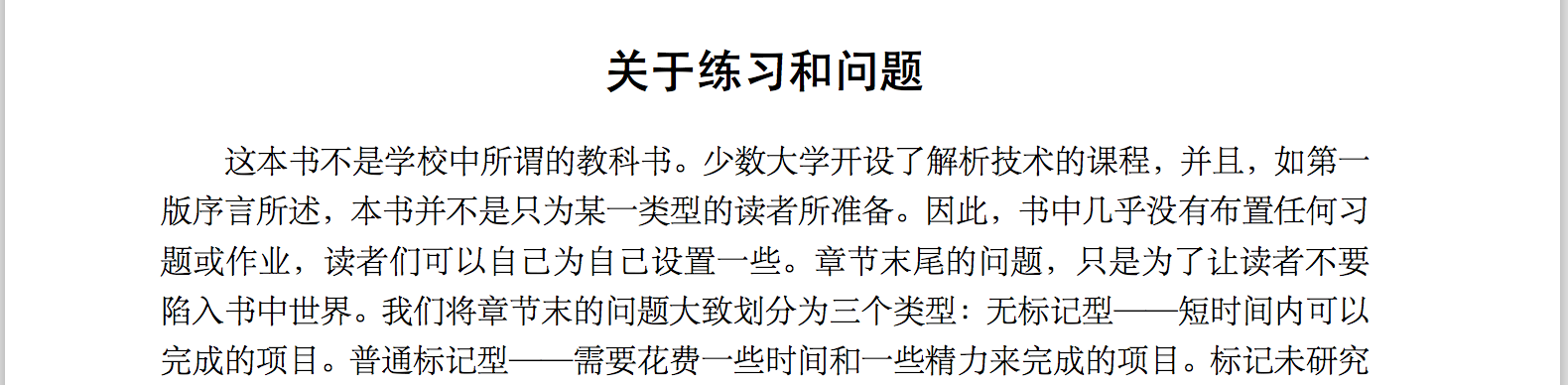• 绘制表格

\begin{figure}[!htbp]
\centering
\begin{tabular}{llp{5cm}ll}
& \textbf{S}      &  &    & \textbf{S}      \\
1b &                 &  & 1b &                 \\
& \textbf{L\&N}   &  &    & \textbf{L\&N}   \\
2a &                 &  & 0c &                 \\
& \textbf{N,L\&N} &  &    & \textbf{L\&h}   \\
0b &                 &  & 2a &                 \\
& \textbf{d,L\&N} &  &    & \textbf{N,L\&h} \\
2b &                 &  & 2b &                 \\
& \textbf{d,N\&N} &  &    & \textbf{N,N\&h} \\
0c &                 &  & 0c &                 \\
& \textbf{d,h\&N} &  &    & \textbf{N,h\&h} \\
0c &                 &  & 0c &                 \\
& \textbf{d,h\&h} &  &    & \textbf{d,h\&h} \\
\end{tabular}
\caption{句子形式变为\textbf{d,h\&h}, 与最左边和最右边替换}
\label{fig:method}
\end{figure}
• 绘制插图

epstopdf fig2_19.eps

\begin{figure}[!htbp]
\centering
\includegraphics[scale=0.6]{figure/fig2_19.pdf}
\caption{Production tree for a context-free grammar}
\label{fig:method}
\end{figure}

• 页面结构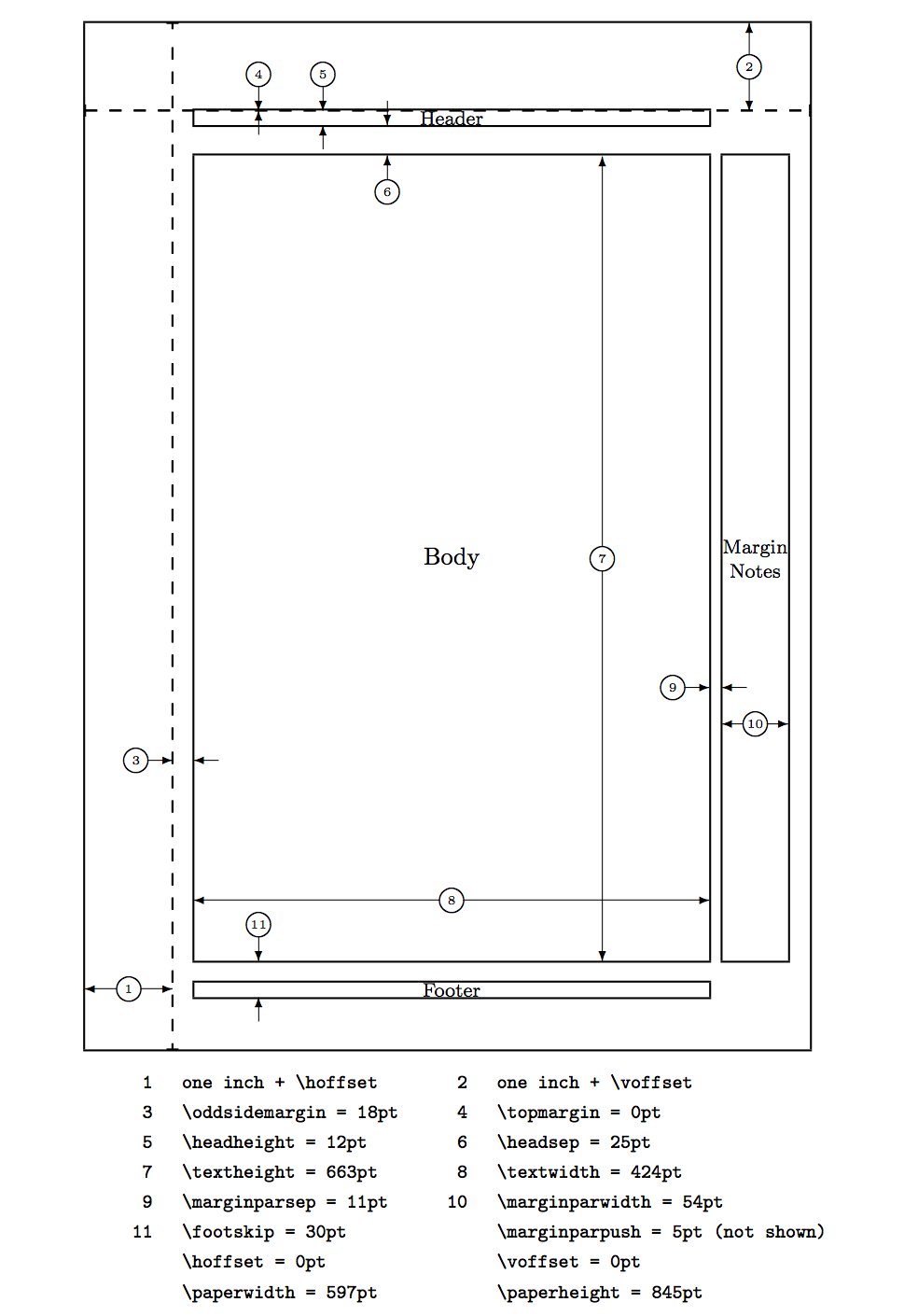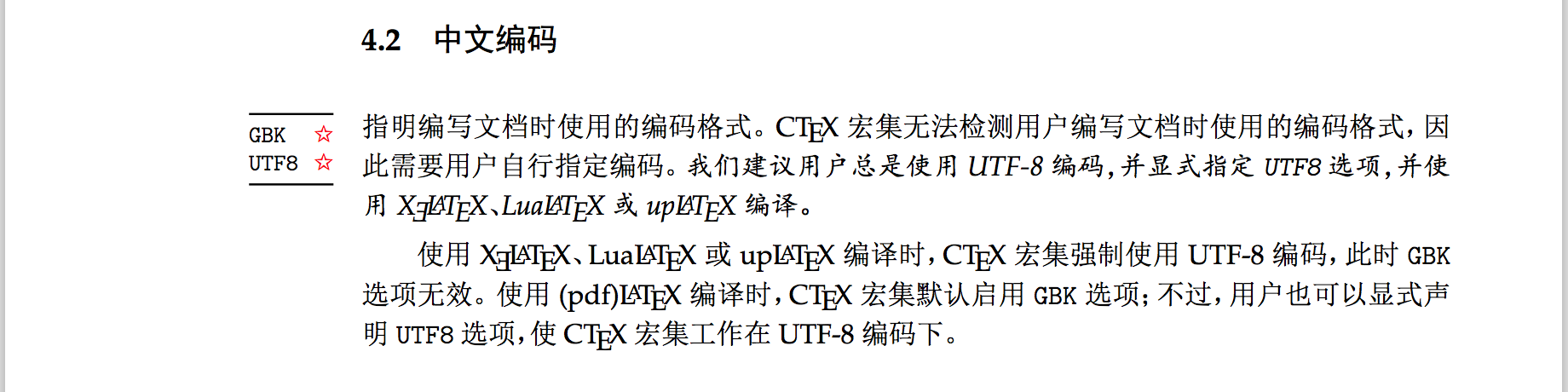\usepackage[left=2.2cm,right=1.8cm,top=2.0cm,bottom=2.5cm]{geometry}
\geometry{papersize={18.00cm,23.00cm}}